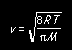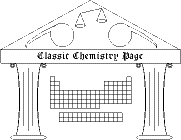## Dalton atomic weights

John Dalton is remembered today for a remarkably fruitful atomic theory. The theory was not correct in every respect; however, it was a pivotal advance in chemistry because it had consequences that could be tested by experiment. Consider a testable prediction based on the following sets of atomic weights: predict the mass ratio of oxygen to nitrogen in "nitrous gas" if the formula is NO.

1a) In the atomic weight scale Dalton began to construct, the atomic weights assigned to nitrogen ("azote") and oxygen were 5 and 7 respectively. Use these atomic weights to predict the O:N mass ratio in NO.
b) Now suppose Dalton had had accurate analyses of water and ammonia available in setting up his system of atomic weights. Dalton decided to use hydrogen as the unit for his system of atomic masses. By weight, the ratio of oxygen to hydrogen in water is 7.94:1 and the ratio of nitrogen to hydrogen in ammonia is 4.63:1. Since Dalton assumed that the formulas of water and ammonia were HO and NH respectively, he would have supposed that the atomic weights of oxygen and nitrogen were 7.94 and 4.63, respectively. Use these atomic weights to predict the O:N mass ratio in NO.
c) Use the atomic weights of nitrogen and oxygen from a modern table to predict the O:N mass ratio in NO. This is the result that an accurate analysis would obtain.

Summary of atomic weights of H, N, and O used in exercise 1.
sourceHNO
a. Dalton 1808157
b. "Daltonesque"14.637.94
c. modern1.00814.00715.999
2) Finally, the chemical analyses to which Dalton had access put the O:N mass ratio in NO between 1.15:1 and 1.36:1 . Should Dalton have seen an inconsistency in the weights for H, N, and O of part 1a? What if he had used the weights of exercise 1b: should he have seen an inconsistency there? Explain.

### Reference

John Dalton, A New System of Chemical Philosophy, Part I, (Manchester, 1808, 1810)
Copyright 2003 by Carmen Giunta. Permission is granted to reproduce for non-commercial educational purposes.Back to the Classic Calculations home pageBack to the top of the Classic Chemistry site